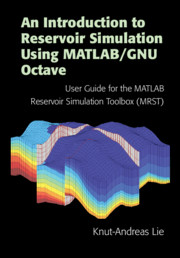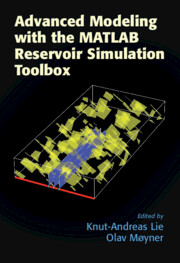# MRST - Transforming research on reservoir simulation

The MRST textbooks
These textbooks are the primary source to learn about models for flow and transport in porous media and how these are implemented in MRST.

#### An Introduction to Reservoir Simulation Using MATLAB/GNU Octave, by Knut-Andreas Lie, Cambridge University Press, 2019, ISBN 9781108492430. URL: www.cambridge.org/9781108492430DOI: 10.1017/9781108591416Buy on Amazon

From the preface: "In this book, I try to (..) give a reasonably self-contained introduction to the simulation of flow and transport in porous media that also discusses how to implement the models and algorithms in a robust and efficient manner. In the presentation, I have tried to let the discussion of models and numerical methods go hand-in-hand with numerical examples that come fully equipped with codes and data, so that you can rerun and reproduce the results by yourself and use them as a starting point for your own research and experiments. (...)

The book can alternatively be seen as a comprehensive user-guide to MRST. (..) This book tries to give an in-depth introduction to MRST and explain the two different programming paradigms you can find in the software. The book is up-to-date with respect to the latest development in data structures and syntax, both for the original procedural approaches that primarily
focus on incompressible flow, as well as for the more recent object-oriented, automatic-differentiation (AD-OO) framework we developed to simulate compressible,
multiphase flow."

The electronic version is freely available as Gold Open Acces, thanks to a generous grant from Equinor.

Scripts and functions necessary for most of the examples can be found in the "book" module of the official MRST release.Citation: K.-A. Lie. An introduction to reservoir simulation using MATLAB/GNU Octave: User guide for the MATLAB Reservoir Simulation Toolbox (MRST). Cambridge University Press, 2019

#### Advanced Modeling with the MATLAB Reservoir Simulation Toolbox, edited by Knut-Andreas Lie and Olav Møyner, Cambridge University Press, 2021, ISBN 9781316519967. URL: www.cambridge.org/core/books/advanced-modeling-with-the-matlab-reservoir-simulation-toolbox/7AC2425C73F6F729DB88DB1A504FA1E7DOI: 10.1017/9781009019781Buy on Amazon

From the preface: "The book you are holding is a follow up to An Introduction to Reservoir Simulation Using MATLAB/GNU Octave published by Cambridge University Press in 2019, which gives a unique introduction on reservoir simulation, with its strong focus on computational aspects and how you would implement all the methods in practice.  (...) In the current book, we expand on the material from the MRST textbook by explaining more recent features in MRST for rapid prototyping and improved computational performance and teaching the mathematical models and computational methods underlying new modules aiming at more accurate and efficient simulations or more advanced reservoir physics. "

The electronic version is freely available as Gold Open Acces, thanks to a generous grant from VISTA, a basic research program funded by Equinor (formerly Statoil) and conducted in close collaboration with the Norwegian Academy of Science and Letters.

Scripts and functions necessary for all the examples can be found in the MRST modules that are associated with each chapter, as described in more detail in the introduction of the book.Citation: Advanced modelling with the MATLAB Reservoir Simulation Toolbox. K.-A. Lie and O. Møyner, eds. Cambridge University Press, 2021

Published August 1, 2019

Publications with MRST

The MRST textbooks

By SINTEF:
By others:
Theses: